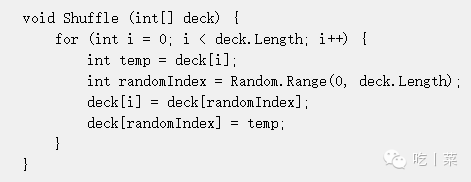# Unity3d学习之随机数的使用

• A+

int Random.Range(int min,int max)

float Random.Range(float min,float max)

Random.value

Vector3(Random.value, Random.value, Random.value)（单位正方形）

Random.insideUnitSphere(单位椭圆)

Random.insideUnitCircle（单位圆）numRequired要取得点的数量

float prob = (float)numToChoose/(float)numLeft;

• 我的微信
• 扫一扫加我微信
•• 微信我的
• 我微信扫一扫加
•